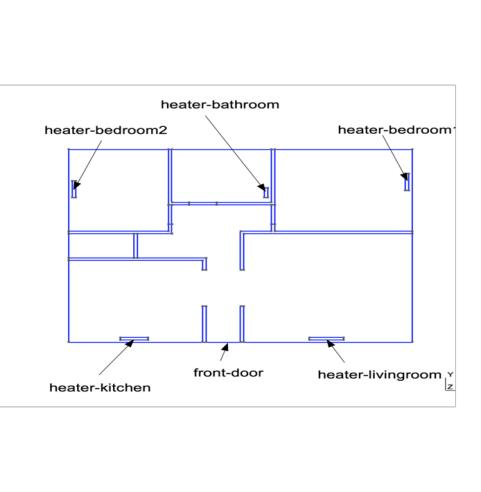# 3D Building

In this example, we estimate an apartment with 5 rooms using the stationary heat equation.

## 1. Running the case

The command line to run this case is

``mpirun -np 4 feelpp_toolbox_heat --case "github:{repo:toolbox,path:examples/modules/heat/examples/3Dbuilding}"``
Case option
`--case "github:{repo:toolbox,path:examples/modules/heat/examples/3Dbuilding}"`

## 2. Data files

The case data files are available in Github here

## 3. GeometryThe mesh can be found on Girder

## 4. Input parameters

Name Description Unit

$k$

thermal conductivity

$W \cdot m^{-1} \cdot K^{-1}$

$T$

temperature

$K$

$T_{ext}$

external temperature

$K$

$h$

transfer coefficient

$W\cdot m^{-2}\cdot K^{-1}$

## 5. Materials

``````    "Materials":
{
"air":
{
"markers":"air",
"k":"1"//[ W/(m*K) ]
//"Cp":"1000.005", //[ J/(kg*K) ]
//"rho":"1.205" //[ kg/(m^3) ]
},
"internal-walls":
{
"markers":"internal-walls",
"k":"0.25"
},
"internal-doors":
{
"markers":"internal-doors",
"k":"0.13"
}
},``````

## 6. Boundary Conditions

The boundary conditions for this thermal probleme are introduced Dirichlet boundary conditions and Robin boundary conditions.

``````    "BoundaryConditions":
{
"temperature":
{
"Dirichlet":
{
"heater-livingroom": { "expr":"325" },
"heater-kitchen": { "expr":"320" },
"heater-bedroom1": { "expr":"315" },
"heater-bedroom2": { "expr":"312" },
"heater-bathroom": { "expr":"298" }
},
"Robin":
{
"exterior-walls":
{
"expr1":"1.0/(0.06+0.01/0.5 + 0.3/0.8 + 0.20/0.032 +0.016/0.313 +0.14)",// h coeff
"expr2":"275"// temperature exterior
},
"front-door":
{
"expr1":"1.0/(0.06+0.06/0.150+0.1/0.029+0.14)", // h coeff
"expr2":"275"// temperature exterior
}
}
}
},``````

## 7. Output

The field of interest for this example is the temperature.

``````    "PostProcess":
{
"Exports":
{
"fields":["temperature"]
}
}``````

From the result of ParaView, we can see that the temperature is dissipated along the 5 heaters.